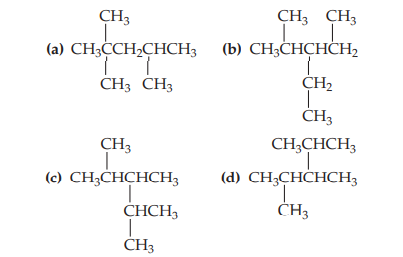×
Get Full Access to Chemistry: The Central Science - 12 Edition - Chapter 24 - Problem 1e
Get Full Access to Chemistry: The Central Science - 12 Edition - Chapter 24 - Problem 1e

×

# Visualizing ConceptsAll the structures shown here have theISBN: 9780321696724 27

## Solution for problem 1E Chapter 24

Chemistry: The Central Science | 12th Edition

• Textbook Solutions
• 2901 Step-by-step solutions solved by professors and subject experts
• Get 24/7 help from StudySoup virtual teaching assistantsChemistry: The Central Science | 12th Edition

4 5 1 309 Reviews
31
5
Problem 1E

All the structures shown here have the molecular formula C₈H₁₈. Which structures are the same molecule? (Hint: One way to answer this question is to determine the chemical name for each structure.) [Section 24.2]n

Step-by-Step Solution:
Step 1 of 3

qll c8 C( \b tAt- irrkr> :CQe -Hr%t "/ rrn r{s ' (-'n^u ,t +14 t-ns s{--Ue >i}r^--hwr so tlneofr^sp^n".^-ht< o', . : ,-l ovnl.l /+\Vo.r,yes t+..44lDsarVtans E t, (f\olr"-\arfi**;a', " Li r.co" 3a-h"r^+ d,rvet\et>,.s' r \Va.n-s i\.\V.o.nzs w ,Ar*'^^laI ' /Vr*e*olu =c-._t-\ .fvlde-.*tu |.t,*Ja'r, ( ,, /z- .,.,nsaL,-^r^-hbn a5 * t F,-rr. A vr a l-Qr*r'^brreJ', A\utz,r g^L tQ

Step 2 of 3

Step 3 of 3

##### ISBN: 9780321696724

Unlock Textbook Solution